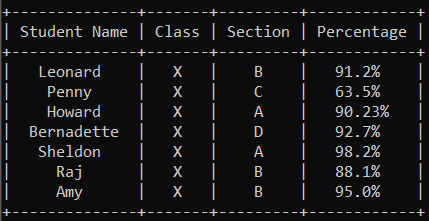Creating Tables with PrettyTable Library – Python

• Last Updated : 18 Aug, 2020

PrettyTable class inside the prettytable library is used to create relational tables in Python. For example, the table below has been created using this library, in Command Prompt on Windows.Installing the Library:

pip install prettytable

Let’s create the sample table using the prettytable library in Python.

Creating the Table: Row-Wise

Python3

 from prettytable import PrettyTable  # Specify the Column Names while initializing the TablemyTable = PrettyTable(["Student Name", "Class", "Section", "Percentage"])  # Add rowsmyTable.add_row(["Leanord", "X", "B", "91.2 %"])myTable.add_row(["Penny", "X", "C", "63.5 %"])myTable.add_row(["Howard", "X", "A", "90.23 %"])myTable.add_row(["Bernadette", "X", "D", "92.7 %"])myTable.add_row(["Sheldon", "X", "A", "98.2 %"])myTable.add_row(["Raj", "X", "B", "88.1 %"])myTable.add_row(["Amy", "X", "B", "95.0 %"])  print(myTable)

Output
+--------------+-------+---------+------------+
| Student Name | Class | Section | Percentage |
+--------------+-------+---------+------------+
|   Leanord    |   X   |    B    |   91.2%    |
|    Penny     |   X   |    C    |   63.5%    |
|    Howard    |   X   |    A    |   90.23%   |
|  Bernadette  |   X   |    D    |   92.7%    |
|   Sheldon    |   X   |    A    |   98.2%    |
|     Raj      |   X   |    B    |   88.1%    |
|     Amy      |   X   |    B    |   95.0%    |
+--------------+-------+---------+------------+

Creating the Table: Column-Wise

Python3

 from prettytable import PrettyTable  columns = ["Student Name", "Class", "Section", "Percentage"]  myTable = PrettyTable()  # Add ColumnsmyTable.add_column(columns, ["Leanord", "Penny", "Howard",                       "Bernadette", "Sheldon", "Raj", "Amy"])myTable.add_column(columns, ["X", "X", "X", "X", "X", "X", "X"])myTable.add_column(columns, ["B", "C", "A", "D", "A", "B", "B"])myTable.add_column(columns, ["91.2 %", "63.5 %", "90.23 %", "92.7 %",                                           "98.2 %", "88.1 %", "95.0 %"])  print(myTable)

Output
+--------------+-------+---------+------------+
| Student Name | Class | Section | Percentage |
+--------------+-------+---------+------------+
|   Leanord    |   X   |    B    |   91.2%    |
|    Penny     |   X   |    C    |   63.5%    |
|    Howard    |   X   |    A    |   90.23%   |
|  Bernadette  |   X   |    D    |   92.7%    |
|   Sheldon    |   X   |    A    |   98.2%    |
|     Raj      |   X   |    B    |   88.1%    |
|     Amy      |   X   |    B    |   95.0%    |
+--------------+-------+---------+------------+

Deleting Rows

myTable.del_row(0)

This will delete the first row from the table, i.e, the rows follow standard indexing starting from index 0.

Clearing the Table

myTable.clear_rows()

This will clear the entire table (Only the Column Names would remain).

There are many advanced features associated with these tables, like converting these tables to HTML or converting a CSV to a PrettyTable. Those functions would be covered in a separate article.

My Personal Notes arrow_drop_up